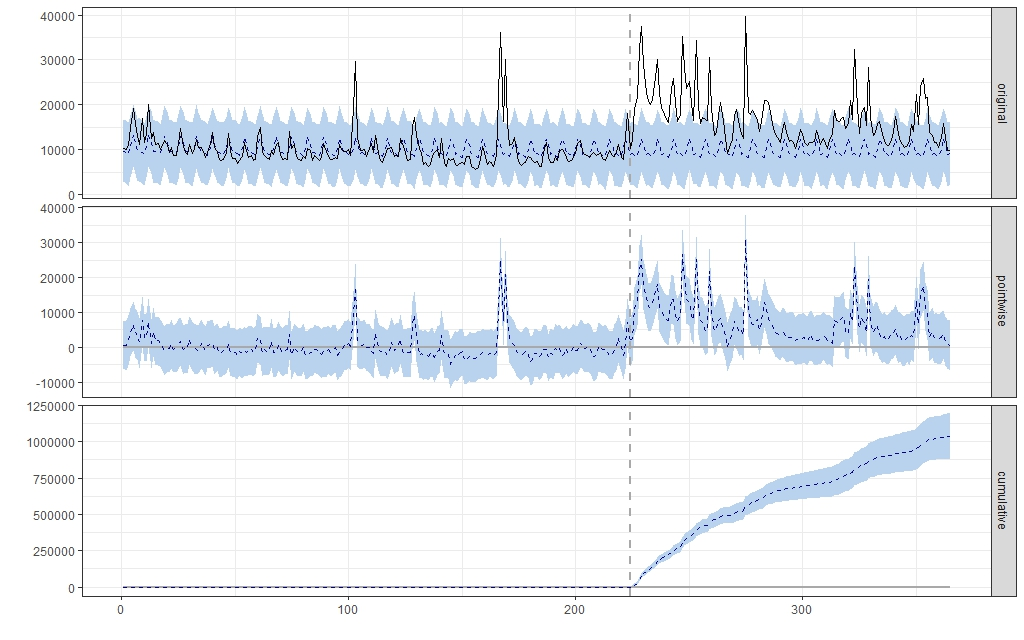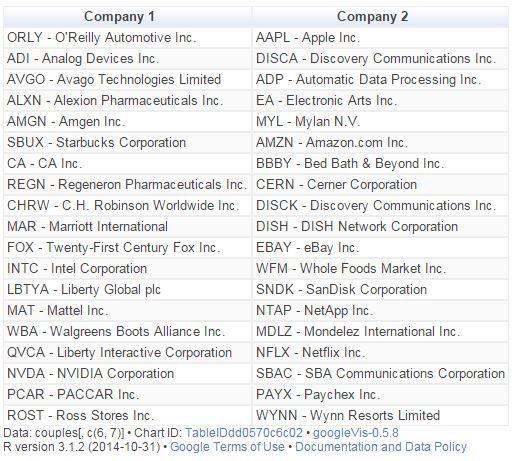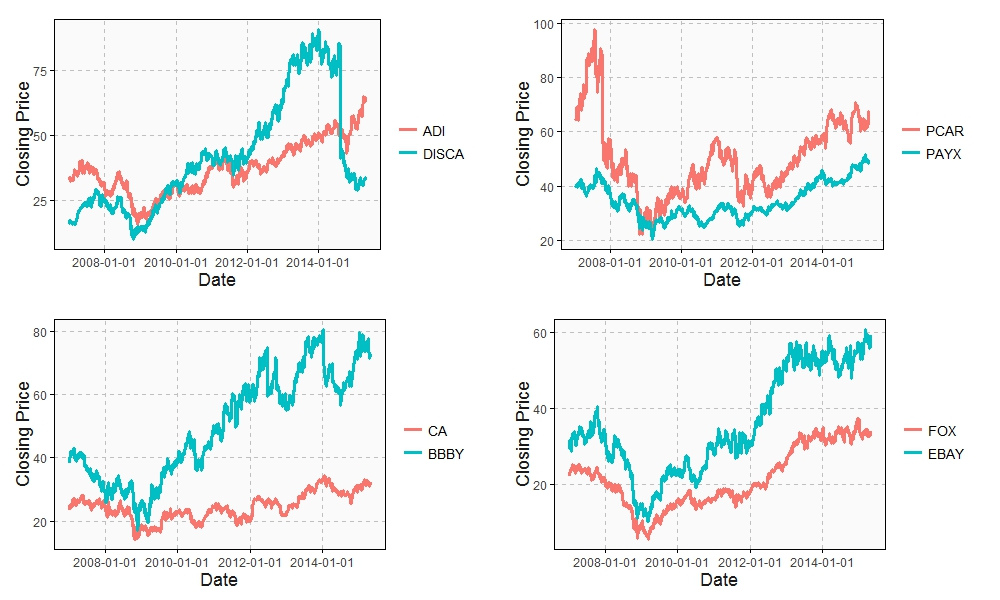# Fatal Journeys: Visualizing the Horror

In war, truth is the first casualty (Aeschylus)

I am not a pessimistic person. On the contrary, I always try to look at the bright side of life. I also believe that living conditions are now better than years ago as these plots show. But reducing the complexity of our world to just six graphs is riskily simplistic. Our world is quite far of being a fair place and one example is the immigration drama.

Last year there were 934 incidents around the world involving people looking for a better life where more than 5.300 people lost their life o gone missing, 60% of them in Mediterranean. Around 8 out of 100 were children.

The missing migrant project tracks deaths of migrants, including refugees and asylum-seekers, who have gone missing along mixed migration routes worldwide. You can find a huge amount of figures, plots and information about this scourge in their website. You can also download there a historical dataset with information of all these fatal journeys, including location, number of dead or missing people and information source from 2015 until today.

I this experiment I read the dataset and do some plots using `highcharter`; you can find a link to the R code at the end of the post.

This is the evolution of the amount of deaths or missing migrants in the process of migration towards an international destination from January 2015 to December 2017:

The Mediterranean is the zone with the most incidents. To see it more clearly, this plot compares Mediterranean with the rest of the world, grouping previous zones:

Is there any pattern in the time series of Mediterranean incidents? To see it, I have done a LOESS decomposition of the time series:

Good news: trend is decreasing for last 12 months. Regarding seasonal component, incidents increase in April and May. Why? I don’t know.

This is a map of the location of all incidents in 2017. Clicking on markers you will find information about each incident:

Every of us should try to make our world a better place. I don’t really know how to do it but I will try to make some experiments during this year to show that we have tons of work in front of us. Meanwhile, I hope this experiment is useful to give visibility to this humanitarian disaster. If someone wants to use the code, the complete project is available in GitHub.

# Who is Alan Turing?

This government is committed to introducing posthumous pardons for people with certain historical sexual offence convictions who would be innocent of any crime now (British Government Spokesperson, September 2016)

Last September, the British government announced its intention to pursue what has become known as the Alan Turing law, offering exoneration to the tens of thousands of gay men convicted of historic charges.  The law was finally unveiled on 20 October 2016.

This plot shows the daily views of the Alan Turing’s wikipedia page during the last 365 days:

There are three huge peaks in May 27th, July 30th, and October 29th that can be easily detected using AnomalyDetection package:After substituting these anomalies by a simple linear imputation, it is clear that the time series has suffered a significant impact since the last days of September:

To estimate the amount of incremental views since September 28th (this is the date I have chosen as starting point) I use CausalImpact package:Last plot shows the accumulated effect. After 141 days, there have been around 1 million of incremental views to the Alan Turing’s wikipedia page (more than 7.000 per day) and it does not seem ephemeral.

Alan Turing has won another battle, this time posthumous. And thanks to it, there is a lot of people that have discovered his amazing legacy: long life to Alan Turing.

This is the code I wrote to do the experiment:

```library(httr)
library(jsonlite)
library(stringr)
library(xts)
library(highcharter)
library(AnomalyDetection)
library(imputeTS)
library(CausalImpact)
library(dplyr)

# Views last 365 days
(Sys.Date()-365) %>% str_replace_all("[[:punct:]]", "") %>% substr(1,8) -> date_ini
Sys.time()       %>% str_replace_all("[[:punct:]]", "") %>% substr(1,8) -> date_fin
url="https://wikimedia.org/api/rest_v1/metrics/pageviews/per-article/en.wikipedia/all-access/all-agents/Alan%20Turing/daily"
paste(url, date_ini, date_fin, sep="/") %>%
GET  %>%
content("text") %>%
fromJSON %>%
.[] -> wikistats

# To prepare dataset for highcharter
wikistats %>%
mutate(day=str_sub(timestamp, start = 1, end = 8)) %>%
mutate(day=as.POSIXct(day, format="%Y%m%d", tz="UTC")) -> wikistats

# Highcharts viz
rownames(wikistats)=wikistats\$day
wikistats %>% select(views) %>% as.xts  %>% hchart

# Anomaly detection
wikistats %>% select(day, views) -> tsdf
tsdf %>%
AnomalyDetectionTs(max_anoms=0.01, direction='both', plot=TRUE)->res
res\$plot

# Imputation of anomalies
tsdf[tsdf\$day %in% as.POSIXct(res\$anoms\$timestamp, format="%Y-%m-%d", tz="UTC"),"views"]<-NA
ts(tsdf\$views, frequency = 365) %>%
na.interpolation() %>%
xts(order.by=wikistats\$day) -> tscleaned
tscleaned %>% hchart

# Causal Impact from September 28th
x=sum(index(tscleaned)<"2016-09-28 UTC")
impact <- CausalImpact(data = tscleaned %>% as.numeric,
pre.period = c(1,x),
post.period = c(x+1,length(tscleaned)),
model.args = list(niter = 5000, nseasons = 7),
alpha = 0.05)
plot(impact)
```

# Odd Connections Inside The NASDAQ-100

Distinguishing the signal from the noise requires both scientific knowledge and self-knowledge (Nate Silver, author of The Signal and the Noise)

Analyzing the evolution of NASDAQ-100 stock prices can discover some interesting couples of companies which share a strong common trend despite of belonging to very different sectors. The NASDAQ-100 is made up of 107 equity securities issued by 100 of the largest non-financial companies listed on the NASDAQ. On the other side, Yahoo! Finance is one of the most popular services to consult financial news, data and commentary including stock quotes, press releases, financial reports, and original programming. Using R is possible to download the evolution of NASDAQ-100 symbols from Yahoo! Finance. There is a R package called `quantmod `which makes this issue quite simple with the function `getSymbols`. Daily series are long enough to do a wide range of analysis, since most of them start in 2007.

One robust way to determine if two times series, xt and yt, are related is to analyze if there exists an equation like yt=βxt+ut such us residuals (ut) are stationary (its mean and variance does not change when shifted in time). If this happens, it is said that both series are cointegrated. The way to measure it in R is running the Augmented Dickey-Fuller test, available in tseries package. Cointegration analysis help traders to design products such spreads and hedges.

There are 5.671 different couples between the 107 stocks of NASDAQ-100. After computing the Augmented Dickey-Fuller test to each of them, the resulting data frame can be converted into a distance matrix. A nice way to visualize distances between stocks is to do a hierarchical clustering. This is the resulting dendogram of the clustering:Close stocks such as Ca Inc. (CA) and Bed Bath & Beyond Inc. (BBBY) are joined with short links. A quick way to extract close couples is to cut this dendogram in a big number of clusters and keep those with two elements. Following is the list of the most related stock couples cutting dendogram in 85 clusters:Most of them are strange neighbors. Next plot shows the evolution closing price evolution of four of these couples:Analog Devices Inc. (ADI) makes semiconductors and Discovery Communications Inc. (DISCA) is a mass media company. PACCAR Inc. (PCAR) manufactures trucks and Paychex Inc. (PAYX) provides HR outsourcing. CA Inc. (CA) creates software and Bed Bath & Beyond Inc. (BBBY) sells goods for home. Twenty-First Century Fox Inc. (FOX) is a mass media company as well and EBAY Inc. (EBAY) does online auctions‎. All of them are odd connections.

This is the code of the experiment:

```library("quantmod")
library("TSdist")
library("ggplot2")
library("Hmisc")
library("zoo")
library("scales")
library("reshape2")
library("tseries")
library("RColorBrewer")
library("ape")
library("sqldf")
library("gridExtra")
setwd("YOUR-WORKING-DIRECTORY-HERE")
temp=tempfile()
for (i in 1:nrow(data)) getSymbols(as.character(data[i,1]))
results=t(apply(combn(sort(as.character(data[,1]), decreasing = TRUE), 2), 2,
function(x) {
ts1=drop(Cl(eval(parse(text=x))))
ts2=drop(Cl(eval(parse(text=x))))
t.zoo=merge(ts1, ts2, all=FALSE)
t=as.data.frame(t.zoo)
m=lm(ts2 ~ ts1 + 0, data=t)
beta=coef(m)
sprd=t\$ts1 - beta*t\$ts2
c(symbol1=x, symbol2=x, (1-ht))}))
results=as.data.frame(results)
colnames(results)=c("Sym1", "Sym2", "TSdist")
results\$TSdist=as.numeric(as.character(results\$TSdist))
save(results, file="results.RData")
m=as.dist(acast(results, Sym1~Sym2, value.var="TSdist"))
hc = hclust(m)
# vector of colors
op = par(bg = "darkorchid4")
plot(as.phylo(hc), type = "fan", tip.color = "gold", edge.color ="gold", cex=.8)
# cutting dendrogram in 85 clusters
clusdf=data.frame(Symbol=names(cutree(hc, 85)), clus=cutree(hc, 85))
clusdf2=merge(clusdf, data[,c(1,2)], by="Symbol")
sizes=sqldf("SELECT * FROM (SELECT clus, count(*) as size FROM clusdf GROUP BY 1) as T00 WHERE size>=2")
sizes2=merge(subset(sizes, size==2), clusdf2, by="clus")
sizes2\$id=sequence(rle(sizes2\$clus)\$lengths)
couples=merge(subset(sizes2, id==1)[,c(1,3,4)], subset(sizes2, id==2)[,c(1,3,4)], by="clus")
couples\$"Company 1"=apply(couples[ , c(2,3) ] , 1 , paste , collapse = " -" )
couples\$"Company 2"=apply(couples[ , c(4,5) ] , 1 , paste , collapse = " -" )
CouplesTable=gvisTable(couples[,c(6,7)])
plot(CouplesTable)
# Plots
opts2=theme(
panel.background = element_rect(fill="gray98"),
panel.border = element_rect(colour="black", fill=NA),
axis.line = element_line(size = 0.5, colour = "black"),
axis.ticks = element_line(colour="black"),
panel.grid.major = element_line(colour="gray75", linetype = 2),
panel.grid.minor = element_blank(),
axis.text = element_text(colour="gray25", size=12),
axis.title = element_text(size=18, colour="gray10"),
legend.key = element_rect(fill = "white"),
legend.text = element_text(size = 14),
legend.background = element_rect(),
plot.title = element_text(size = 35, colour="gray10"))
plotPair = function(Symbol1, Symbol2)
{
getSymbols(Symbol1)
getSymbols(Symbol2)
close1=Cl(eval(parse(text=Symbol1)))
close2=Cl(eval(parse(text=Symbol2)))
cls=merge(close1, close2, all = FALSE)
df=data.frame(date = time(cls), coredata(cls))
names(df)[-1]=c(Symbol1, Symbol2)
df1=melt(df, id.vars = "date", measure.vars = c(Symbol1, Symbol2))
ggplot(df1, aes(x = date, y = value, color = variable))+
geom_line(size = I(1.2))+
scale_color_discrete(name = "")+
scale_x_date(labels = date_format("%Y-%m-%d"))+
labs(x="Date", y="Closing Price")+
opts2
}
p2=plotPair("PCAR", "PAYX")
p3=plotPair("CA", "BBBY")
p4=plotPair("FOX", "EBAY")
grid.arrange(p1, p2, p3, p4, ncol=2)
```

# NASDAQ 100 Couples

Heaven, I’m in heaven, and my heart beats so that I can hardly speak, and I seem to find the happiness I seek, when we’re out together dancing cheek to cheek (Cheek To Cheek, Irving Berlin)

There are about 6.500 available packages in CRAN repository. If I were a superhuman, able to learn one package a day, I would spend almost 18 years of my life studying R. And how many packages would be uploaded to CRAN during this period? Who knows: R is infinite.

Today, my experiment deals with `quantmod` package, which allows you to play to be quant for a while. I download the daily quotes of NASDAQ 100 companies and measure distances between each pair of companies. Distance is based on the cross-correlation between two series so high-correlated series (not exceeding a maximum lag) are closer than low-correlated ones. You can read a good description of this distance here. Since NASDAQ 100 contains 107 companies, I calculate distances for 5.671 different couples. Next plot represent distances between each pair of companies. The darker is the color, the closer are the related companies:Yes, I know is not a graph for someone with visual problems. Let me show you an example of what is behind one of these little tiles. Distance between Mattel Inc. and 21st Century Fox is very small (its related tile is dark coloured). Why? Because of this:These two companies have been dancing cheek to cheek for more than seven years. It is also curious how some companies are far from any of their NASDAQ 100 colleagues. Some examples of these unpaired companies are Express Scripts Holding Company (ESRX), Expeditors International of Washington Inc. (EXPD) and Fastenal Company (FAST). I do not why but there must be an explanation, do not you think so?

Something tells me I will do some other experiment using quantmod package:

```library("quantmod")
library("TSdist")
library("ggplot2")
library("Hmisc")
library("zoo")
library("scales")
library("reshape2")
temp=tempfile()
for (i in 1:nrow(data)) getSymbols(as.character(data[i,1]))
results=t(apply(combn(sort(as.character(data[,1]), decreasing = TRUE), 2), 2,
function(x)
{
ts1=drop(Cl(eval(parse(text=x))))
ts2=drop(Cl(eval(parse(text=x))))
c(symbol1=x, symbol2=x, tsDistances(ts1, ts2, distance="crosscorrelation"))
}))
results=as.data.frame(results)
colnames(results)=c("Sym1", "Sym2", "TSdist")
results\$TSdist=as.numeric(as.character(results\$TSdist))
results=rbind(results, data.frame(Sym1=as.character(data[,1]), Sym2=as.character(data[,1]), TSdist=0))
results\$TSdist2=as.numeric(cut2(results\$TSdist, g=4))
opts=theme(axis.text.x = element_text(angle = 90, vjust=.5, hjust = 0),
panel.background = element_blank(),
axis.text = element_text(colour="gray25", size=8),
legend.position = "none",
panel.grid = element_blank())
ggplot(results,aes(x=Sym2,y=Sym1))+
geom_tile(aes(fill = TSdist2), colour="gray80")+
scale_size_continuous(range=c(1,10))+
scale_x_discrete("", limits=sort(unique(as.character(results\$Sym1))))+
scale_y_discrete("", limits=sort(unique(as.character(results\$Sym2)), decreasing = TRUE))+
scale_fill_gradient(low = "steelblue", high = "white")+
opts
MAT.close=Cl(MAT)
FOX.close=Cl(FOX)
cls=merge(MAT.close, FOX.close, all = FALSE)
df=data.frame(date = time(cls), coredata(cls))
names(df)[-1]=c("mat", "fox")
df1=melt(df, id.vars = "date", measure.vars = c("mat", "fox"))
opts2=theme(
panel.background = element_rect(fill="gray98"),
panel.border = element_rect(colour="black", fill=NA),
axis.line = element_line(size = 0.5, colour = "black"),
axis.ticks = element_line(colour="black"),
panel.grid.major = element_line(colour="gray75", linetype = 2),
panel.grid.minor = element_blank(),
axis.text = element_text(colour="gray25", size=15),
axis.title = element_text(size=18, colour="gray10"),
legend.key = element_blank(),
legend.position = "none",
legend.background = element_blank(),
plot.title = element_text(size = 40, colour="gray10"))
ggplot(df1, aes(x = date, y = value, color = variable))+
geom_line(size = I(1.2))+
scale_color_discrete(guide = "none")+
scale_x_date(labels = date_format("%Y-%m-%d"))+
labs(title="Nasdaq 100 Couples: Mattel And Fox", x="Date", y="Closing Price")+
annotate("text", x = as.Date("2011-01-01", "%Y-%m-%d"), y = c(10, 30), label = c("21st Century Fox", "Mattel Inc."), size=7, colour="gray25")+
opts2
```